CalculusShowing results 31 to 40 of 52, on page 4 of 6 Differentiation/Differentals Integration/Integrals Limits Sequences and Series
 DESCRIPTION EQUATION Finite Geometric Series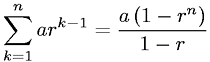Fundamental Theorem for Derivatives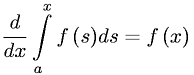Fundamental Theorem of Integrals of Derivatives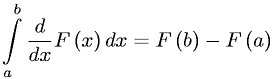Infinite Geometric Series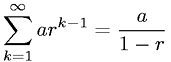Integral of cosecant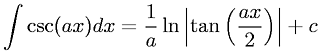Integral of cosineIntegral of powers not equal to -1Integral of reciprocal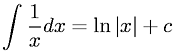Integral of secant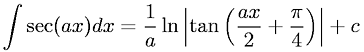Integral of sine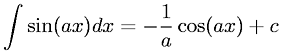Go to page: 1  2  3  4  5  6Terms of Service Add to Favorites Contact Us © 2012 EquPlus.net